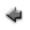previous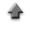home

# Histogram

You can draw a histogram. To start, click "Histogram" tab on the application.
If you use the sample data, click here, and save it in the appropriate directory (or folder).

When you draw the histogram of the sample data,

1. Click "Open data file", and specify the data file name saved according to the above instruction.
2. When clicking the "Histogram" button bellow, the histogram is displayed.

## Data

1. Each label for data can be set in the first row in the data file. The label can not include spaces. Each label has to be delimited by space or tab.
2. Data must be inputted by normal width.
3. Input a numerical datum a line in the data file, change the line, and input the datum of the following row similarly until the input of the last datum ends. Refer to the following examples, where x1, x2,...,xn are the numerical data.
 x1 x2 . . . xn
4. In addition, you can use the table data form in the data file. You may include the column data in the data file which are not used to make a histogram. In that case, the specification of the column in the data file used to make the histogram is needed at the line "Data Columns" in the following "Input" instruction.

## Inputs

1. Click "Open data file" and specify the data file prepared according to the above instruction.
2. When the labels for data are used, check the "Data labels" box.
3. The column in the data file to be used to make a histogram can be specified at the line "Data columns". For instance, when the data to make the histogram are put at the fifth column in the data file, specify "5" at the line "Data columns", and check "Data columns" box.
4. The range for the histogram can be specified at the line "Data range". In that case, specify the range for the histogram (the minimum and the maximum values must be delimited by space), and check the "Data range" box. For instance, when you want to assume the range for the histogram to be 0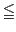x15, specify "0 15" at the line "Data range", and check the "Data range" box.
5. The number of channels in the histogram can be specified at the line "Number of channels". When the number of channels has been specified, and the "Number of channels" box at the line must be checked. By default, the bin width is set as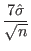, where,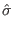is the sample standard deviation and n is the number of data.
6. The value of the weight for the histogram can be changed at the line "Weight", if necessary. When the value of the weight has been changed, the "Weight" box at the line must be checked.
7. The class interval in the histogram can be set at the line "Intervals" arbitrarily . Specify the values of the following class delimiting by space from the minimum value of the class to the maximum value one by one, and check the "Intervals" box. In this case, the setting of the number of channels at the line "Number of channels" becomes invalid. Moreover, when the range for the histogram at the line "Data range" is set at the same time, the priority is given to the setting at the line "Intervals".
8. The title for the histogram can be specified at the line "Graph title". Click the "Set" button after describing it.
9. The label of the abscissa in the histogram can be specified at the line "Label of abscissa". Click the "Set" button after describing it.
10. The label of the ordinate in the histogram can be specified at the line "Label of ordinate". Click the "Set" button after describing it.
11. The explanatory note (legend) of the histogram can be specified at the line "Legend of data". Click the "Set" button after describing it.

## Outputs

1. When "Show histogram" button is clicked, the histogram is displayed.
2. When the "Show frequency table summary" button is clicked, the summary of the histogram is displayed.
3. When the "Show frequency table" button is clicked, the frequency table is displayed.
4. When the "Show cumulative frequency" button is clicked, the histogram with the cumulative frequeny can be displayed also.
5. When the "Show data summary" button is clicked, the statistics of the data used to make the histogram is displayed.

## Fitting a function to the histogram

You can fit a function model to the histogram.
• For maximum likelihood fitting, the value of x (abscissa) is set to class mark at each class interval. The value of y (ordinate) is set to class frequency n at each class interval.
• For least squares fitting, the value of x (abscissa) is set to class mark at each class interval. The value of y (ordinate) is set to class frequency n and the error of the ordinate to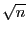at each class interval.
1. Input a function model to be fitted at the line "Function f(x)", and click the "Set" button. About the input of the function, refer here.
2. This fitting algorithm requires the specification of initial values of the parameters included in the function model. Then, you must input the initial values of the parameters at the line "Parameters" which must be delimited by space, and click the "Set" button.
3. The explanatory note (legend) of the function can be specified at the line "Legend". Click the "Set" button after describing it.
4. When you use the second order derivatives of the function with respect to the parameters in computation of the curvature matrix elements and the parameter's errors, check the "2nd order derivatives" box.
5. A result of fitting can be displayed when "Maximum likelihood fit" or "Least squares fit" button is clicked, and the fitted function is also drawn in the graph.
6. When the "Show error matrix" button is clicked, the elements of the error matrix is displayed.
7. When the "Show residual graph" button is clicked, the plot of the residuals is displayed.
8. When the "Show residuals" button is clicked, the data of the residuals is displayed.

Kazushi Neichi